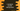# C program to print half right angled triangle or half pyramid# C program to print half pyramid or half right angled triangle :

In this C programming tutorial, we will learn how to print a half pyramid or half right angled triangle using a special character or any other character. In this tutorial, we will learn how to print a half right angled triangle :

1. With any special character
2. With incrementing digits from start to end.
3. With incrementing digits on each line starting from 1.
4. With different characters on each line.

## With any special character :

The C program will look like below :

``````#include <stdio.h>
int main()
{
//1
int i, j, row;
char c;
//2
printf("Enter total number of rows for the pyramid : ");
scanf("%d", &row);
//3
printf("Enter the character to use : ");
scanf(" %c", &c);
//4
for (i = 1; i <= row; ++i)
{
//5
for (j = 1; j <= i; ++j)
{
printf("%c ", c);
}
printf("\n");
}
return 0;
}``````

### Explanation :

The commented numbers in the above program denote the step numbers below :

1. Create three integers i,j and row. i and j will be used in loops and row are to store the total row count. Also, create one character c to store the character for printing the pyramid.
2. Ask the user to enter the total number of rows for the pyramid. Read it and store it in the row variable.
3. Ask the user to enter the character to use for printing the pyramid. Read it and store in the c variable.
4. Run one for loop. This loop will run for row amounts of time. If we are printing one pyramid with 4 rows, it will run for 4 times.
5. Run one inner for loop. This inner for loop is used to print the characters for each row. For the first row, we will print 1 character, for the second row, 2 characters etc. So, this loop will run for the same as the value of i. Inside this loop, print the character.

### Sample Output :

``````Enter total number of rows for the pyramid : 5
Enter the character to use : *
*
* *
* * *
* * * *
* * * * *

Enter total number of rows for the pyramid : 8
Enter the character to use : @
@
@ @
@ @ @
@ @ @ @
@ @ @ @ @
@ @ @ @ @ @
@ @ @ @ @ @ @
@ @ @ @ @ @ @ @``````

## With incrementing digits from start to end :

``````#include <stdio.h>
int main()
{
int i, j, row;
int flag = 1;
printf("Enter total number of rows for the pyramid : ");
scanf("%d", &row);
for (i = 1; i <= row; ++i)
{
for (j = 1; j <= i; ++j)
{
printf("%d ", flag);
flag++;
}
printf("\n");
}
return 0;
}``````

### Sample Output :

``````Enter total number of rows for the pyramid : 5
1
2 3
4 5 6
7 8 9 10
11 12 13 14 15

Enter total number of rows for the pyramid : 8
1
2 3
4 5 6
7 8 9 10
11 12 13 14 15
16 17 18 19 20 21
22 23 24 25 26 27 28
29 30 31 32 33 34 35 36

Enter total number of rows for the pyramid : 2
1
2 3``````

## With incrementing digits on each line :

``````#include <stdio.h>
int main()
{
int i, j, row;
int flag;
printf("Enter total number of rows for the pyramid : ");
scanf("%d", &row);
for (i = 1; i <= row; ++i)
{
flag = 1;
for (j = 1; j <= i; ++j)
{
printf("%d ", flag);
flag++;
}
printf("\n");
}
return 0;
}``````

### Sample Output :

``````Enter total number of rows for the pyramid : 5
1
1 2
1 2 3
1 2 3 4
1 2 3 4 5

Enter total number of rows for the pyramid : 7
1
1 2
1 2 3
1 2 3 4
1 2 3 4 5
1 2 3 4 5 6
1 2 3 4 5 6 7

Enter total number of rows for the pyramid : 3
1
1 2
1 2 3``````

## With different character on each line :

``````#include <stdio.h>
int main()
{
int i, j, row;
char c = 'a';
printf("Enter total number of rows for the pyramid : ");
scanf("%d", &row);
for (i = 1; i <= row; ++i)
{
for (j = 1; j <= i; ++j)
{
printf("%c ", c);
}
c++;
printf("\n");
}
return 0;
}``````

### Sample Output :

``````Enter total number of rows for the pyramid : 5
a
b b
c c c
d d d d
e e e e e

Enter total number of rows for the pyramid : 7
a
b b
c c c
d d d d
e e e e e
f f f f f f
g g g g g g g

Enter total number of rows for the pyramid : 3
a
b b
c c c``````